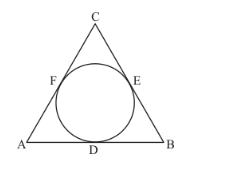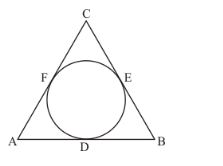# In the adjacent figure, If AB = 12 cm, BC = 8 cm and AC = 10 cm, then AD =

Question:

In the adjacent figure, If AB = 12 cm, BC = 8 cm and AC = 10 cm, then AD =(a) 5 cm

(b) 4 cm

(c) 6 cm

(d) 7 cm

Solution:

By looking at the given figureWe can write the following equations:

We know that tangents form an external point will be of equal length. Therefore,

DB = BE …… (1)

Hence we have,

From the figure, we have

BE + EC = 8 …… (2)

Let us subtract equation (2) from equation (3). We get,

AD − EC = 4 …… (3)

Since tangents from an external point will be equal, we have,

EC = CF

Therefore, equation (3) becomes,

AF − CF = 4 …… (4)

From the figure we have,

AF + CF = 10 …… (5)

Adding equation (4) and (5), we get,

2AF = 14

AF = 7

We know that,